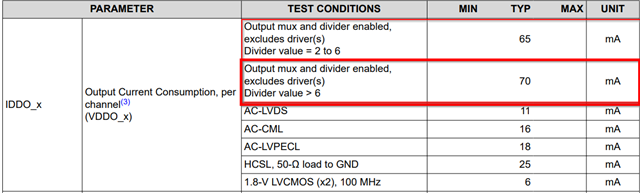If you have a related question, please click the "Ask a related question" button in the top right corner. The newly created question will be automatically linked to this question.

# LMK05318: Power Consumption Calculation

Part Number: LMK05318

Hi :

Datasheet, power consumption example, page 75:

1) Why there is 70mA in power consumption calculation in page 75 of datasheet, for every output?

IDDO_01 = 70 + 16 + 16 = 102 mA
• IDDO_23 = 70 + 14 + 14 = 98 mA
• IDDO_4 = 70 + 10 = 80 mA
• IDDO_5 = 0 mA
• IDDO_6 = 70 + 25 = 95 mA
• IDDO_7 = 70 + 6 = 76 mA

2) If all output frequencies are the same, same divider scale, how 70mA would change in above datasheet example?

3) Is there a SW tool by TI to estimate power consumption of LMK05318, based on design settings ?

Thanks,

K.

• Hi K.,

I will look into this question 1) and 2).

For 3), we do not have a power consumption calculator available at the moment.

Regards,

Jennifer

• Hi K.,

In the datasheet example, every output has 70 mA for power consumption because they use a divider value >6. This number is a typical measurement and can be found in the electrical characteristics table. You can expect similar performance for outputs using divider values from 2 to 6 (65 mA, typ.) or outputs using greater than 6 (70 mA, typ.).Regards,

Jennifer